Williams' AD (Wad) is the accumulated sum of positive "accumulational" and negative "distributional" price movements.  For example, if the current closing price is higher than the previous one, Wad increases by the difference between the current closing price and the true minimum.  If the current closing price is lower than the previous one, Wad decreases by the difference between the current closing price and the true maximum.Market Signals

If the price reaches a new minimum, but the accumulation/distribution indicator cannot reach a new minimum, it means that the security is accumulating.  This is a buy signal.  If the price reaches a new maximum, but the accumulation/distribution indicator cannot reach a new maximum, it means that the security is distributing itself.  This is a sell signal.Calculation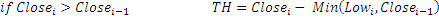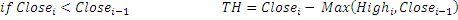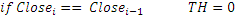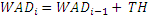Chart Example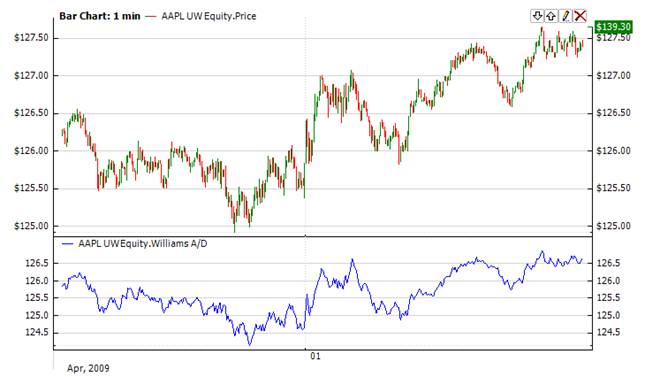Implementation and Usage

Indicator has no input parameters. To initialize Wad indicator use the following constructor:

Use

WAD - property to get current valueExample
C#
``` 1// Create new instance
3
4// Number of stored values